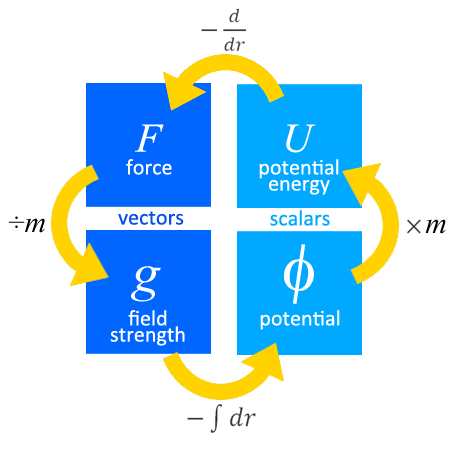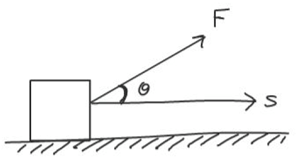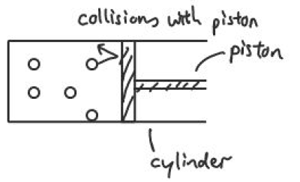## 07. Gravitation

[spoiler title="Gravitational Field" open="yes"]

• Newton’s Law of Gravitation states that the gravitational force between two point masses is directly proportional to the product of their masses and inversely proportional to the square of their separation,
i.e. $F_G=\dfrac{Gm_1m_2}{r^2}$ where G is the gravitational constant 6.67 $\times$ 10-11 N kg-2m2, m1 and m2 are the masses and r is the distance between them.
• Gravitational field strength at a point is defined as the gravitational force acting per unit mass placed at that point,
i.e. $g=\dfrac{F_G}{m_2}=\dfrac{Gm_1}{r^2}$ where m1 is the mass of the source of the field.
• Gravitational potential energy at a point is defined as the work done by an external agent in bringing a body from infinity to that point (without any change in kinetic energy),
i.e. $U=-\dfrac{Gm_1m_2}{r}$
• Gravitational potential at a point is defined as the work done per unit mass by an external agent in bringing a body from infinity to that point (without any change in kinetic energy),
i.e. $V=-\dfrac{Gm_1}{r}$[/spoiler]

[spoiler title="Interplanetary Travel"]

• Escape speed is the minimum speed at which a body of mass m can be projected from the surface of a planet of mass M so that it reaches an infinite distance from the planet.
• By conservation of energy,
initial total energy = final total energy
$U_i+E_{k_i}=U_f+E_{k_f}\\-\dfrac{GMm}{r}+\dfrac{1}{2}mv^2=0+0$
$v=\sqrt{2GM}$

[/spoiler]

[spoiler title="Satellite Motion"]

• For an object with mass m in orbit,
Gravitational Force = Centripetal Force
$F_G=F_C\\\dfrac{GMm}{r^2}=mr\omega^2\\\dfrac{GM}{r^2}=r(\dfrac{2\pi}{T})^2\\\text{period, }T=2\pi\sqrt{\dfrac{r^3}{GM}}$
• $F_G=F_C\\\dfrac{GMm}{r^2}=\dfrac{mv^2}{r}\\\text{orbital speed, }v=\sqrt{\dfrac{GM}{r}}$
• kinetic energy, $E_k=\dfrac{1}{2}mv^2=\dfrac{GMm}{2r}$
• total energy, $E_T=E_k+U=\dfrac{GMm}{2r}-\dfrac{GMm}{r}=-\dfrac{GMm}{2r}$

[/spoiler]

[spoiler title="Geostationary Satellites"]

• A geostationary satellite (one that is always above the same point on the Earth) has to be in an equatorial orbit, i.e. the orbit is in the same plane as that of the equator, so that
• the centripetal force points towards the centre of the plane, and
• it moves in the same direction of rotation as the Earth, i.e. from west to east.
• It has a period of 24 h, i.e. same period as the Earth.
• It orbits with a fixed radius of 4.20 $\times$ 10m from the Earth's centre.

[/spoiler]

## 06. Motion in a Circle

[accordions autoHeight='true']

[accordion title="1. Rotational Kinematics"]

• Angular displacement $\theta$ is defined as the angle an object turns with respect to the centre of a circle. $\theta=\dfrac{s}{r}$ where s is the arc and r is the radius of the circle.
• One radian is the angular displacement when the arc length is equal to the radius of the circle.
• Angular velocity $\omega$ is defined as the rate of change of angular displacement. $\omega=\dfrac{d\theta}{dt}$
• For motion in a circle of fixed radius, $\omega=\dfrac{d\theta}{dt}=\dfrac{d(\dfrac{s}{r})}{dt}=\dfrac{1}{r}\dfrac{ds}{dt}=\dfrac{v}{r}$. Thus $v=r\omega$.
• Average angular velocity in one cycle. $\omega=\dfrac{2\pi}{T}=2\pi f$ where T is the period and f is the frequency.

[/accordion]

[accordion title="2. Centripetal Force"]

• Centripetal acceleration $a=\dfrac{v^2}{r} = r\omega^2$.
• Centripetal force $F =\dfrac{mv^2}{r} = mr\omega^2$.

[/accordion]

[accordion title="3. Uniform Circular Motion"]

For a body in uniform circular motion, there is a change in velocity as the direction of motion is changing. This requires an acceleration that is perpendicular to the velocity and directed towards the centre of circle. This acceleration is provided by a centripetal force. A resultant force acting on a body toward the centre of a circle provides the centripetal force.

[/accordion]

[accordion title="4. Non-Uniform Circular Motion"]

Learn how to solve problems on circular motions of conical pendulum, cyclist, car, aircraft, swinging a pail etc.

[/accordion]

[/accordions]

## 05. Work, Energy and Power

[accordions autoHeight='true']

[accordion title="1. Work Done"]

• Work done W is defined as the product of the force and the displacement made in the direction of the force.
• For a constant force F acting in same direction as the displacement s: W = F . s
• If $\theta$ is the angle between F and s:  $W = F\cos\theta\cdot s$• Work done = Area under F-s graph.[/accordion]

[accordion title="1.1 Work Done on a Spring"]

• Work done in stretching a spring = area under F-x graph = ½ Fx = ½ kx2[/accordion]

[accordion title="1.2 Work Done by a Gas"]

• Work done by expanding gas = area under P-V graph = $\int P.dV$[/accordion]

[accordion title="2. Kinetic Energy"]

• Kinetic energy is the energy of a body by virtue of its motion.
• Derive kinetic energy: W = F.s = ma.s = m ½(v2 – u2)

[/accordion]
[accordion title="3. Potential Energy"]

• Potential energy is the energy of a body by virtue of it position.
• Gravitational Potential Energy near Earth’s surface: $E_p = mgh$[/accordion]

[accordion title="4. Conservation of Energy"]

• The Principle of Conservation of Energy states that energy can neither be created nor destroyed in any process. It can be transformed from one form to another, and transferred from one body to another but the total amount remains constant.
• The Work-Energy Theorem states that the net work done by external forces acting on a particle is equal to the change in mechanical energy of the particle. i.e. $W=\Delta E_k+\Delta E_p$
• Force $F =-\dfrac{dU}{dx} = -\text{(gradient of Potential Energy vs displacement graph)}$

[/accordion]

[accordion title="5. Power"]

• Power is work done per unit time
• Instantaneous Power: $P = \dfrac{dW}{dt} = F \dfrac{ds}{dt} = F v$,
• Average Power: $

= \dfrac{W}{t} = F $

[/accordion]

[accordion title="6. Efficiency"]

• Efficiency $\eta= \dfrac{E_{output}}{E_{input}}\times 100 \% \text{ or} \dfrac{P_{output}}{P_{input}}\times100\%$

[/accordion]
[/accordions]

## Electromagnetic Induction and its Effects

To explain a phenomenon that happens due to Electromagnetic Induction, we can use an acronym CFILE to structure our answer.

C stands for cutting of flux or changing of flux linkage. This is necessary for electromagnetic induction to happen. When a wire is pulled through a magnetic field perpendicular to it, it is said to be cutting the field lines. When a magnet enters a coil of wires, we can say that the magnetic flux linkage is increasing.

F stands for Faraday's law, which states that the induced e.m.f. in a circuit is directly proportional to the rate of change of flux-linkage or to the rate of cutting of magnetic flux.

I stands for an induced current. However, do note that this is only possible if there is a closed circuit or a path for the current to flow.

L stands for Lenz's law, which states that the direction of the induced e.m.f. is such that it tends to oppose the flux change causing it, and does oppose it if induced current flows

E stands for effect. This is really just stating how an induced current or Lenz's law causes the phenomenon in question.

## 17. Alternating Currents

• The root-mean-square value of an alternating current is equivalent to the steady direct current that would dissipate heat at the same rate as the alternating current in a given resistor.
• For a sinusoidal source,
(a) the root mean square value of the current is given by $I_{rms}=\frac{I_o}{\sqrt{2}}$.
(b) the mean or average power < P > absorbed by a resistive load is half the maximum power.
$

=\frac{1}{2}P_o=\frac{1}{2}{I_o}{V_o}=\frac{1}{2}{I_o}^2R =\frac{V_o^2}{2R}$

.
• An a.c. transformer is a device for increasing or decreasing an a.c. voltage. It consists of a primary coil of Np turns and voltage Vp and secondary coil of Ns turns and voltage Vs wrapped around an iron core.
• For an ideal transformer (assuming no energy is lost), the following equation is obeyed
$\frac{N_s}{N_p}=\frac{V_s}{V_p}=\frac{I_p}{I_s}$.
• Power loss in the transmission lines is minimized if the power is transmitted at high voltages (i.e. low currents) since $P_{loss}=I^2R$ where I is the current through the cables and R is the resistance of the cables.
• The equation $P=\frac{V^2}{R}$ is often mistakenly used to suggest that power lost is high when voltage of transmission is high. In fact, V refers to the potential difference across the cables, which often have but a fraction of the overall resistance through which the current passes.

## 18. Quantum Physics

[accordions autoHeight='true']

[accordion title="1. Particle Nature of Light"]

• photon is a quantum of electromagnetic radiation.
• The energy of a photon is given by E=hf, where h is Planck's constant (6.63 $\times$ 10-34 J s) and f is its frequency.

[/accordion]

[accordion title="1.1 Photoelectric Effect"]

• The photoelectric effect is the emission of electrons from a metal surface when electromagnetic radiation of sufficiently high frequency is shone on it.
• The energy of an incident photon is the sum of the maximum kinetic energy $K.E._{max}$ of the emitted electrons from the metal surface and the work function $\Phi$ of the metal. Einstein's photoelectric equation states that

$hf=\Phi +K.E._{max}=hf_o +K.E._{max}$

• where $f_o$ is the threshold frequency or minimum frequency of the electromagnetic radiation below which no electrons are emitted from the metal surface regardless of the intensity of the radiation.
• The work function $\Phi$ of a metal is the minimum energy needed to remove an electron from the metal surface.
• $K.E._{max}$ can be measured by applying a voltage to prevent the emitted electrons from reaching the electrode that collects them. This voltage is known as the stopping voltage $V_s$ and since the charge of an electron is e, the equation can be rewritten as

$hf=\Phi + eV_s$.

[/accordion]

[accordion title="1.2 Line Spectra"]

• An atom is in the ground state when its electron occupies the lowest energy level. When the atom gains energy, its ground state electron makes a transition to a higher energy level. The atom is said to be in an excited state.
• At this excited state, the electron is unstable. It will jump to a lower energy level by emitting a photon whose energy is equal to the energy difference between the two levels. The photon energy is given hf = Ehigher – Elower.
• The emission line spectra are the spectra of light radiated by individual atoms in a hot gas when the electrons in the atoms jump from higher energy levels to lower energy levels. Each spectrum consists of coloured lines on a dark background.
• The absorption line spectra consists of dark lines on a coloured background. When a beam of white light is passed through a cool gas, photons whose energies are equal to the excitation energies of the gas atoms, are absorbed. These photons are re-emitted in all directions, so the intensity of these wavelengths in the transmitted white light beam is reduced.

[/accordion]

[accordion title="2. Wave Nature of Particles"]

• Louis de Broglie postulated that, because photons have wave and particle characteristics, perhaps all forms of matter have both properties. Electron diffraction provides evidence for the wave nature of particles.
• The de Broglie wavelength of a particle is given by $\lambda = \dfrac{h}{p}$ where p is the momentum (mv) of the particle and h is Planck’s constant.

[/accordion]

[accordion title="3. X-ray Spectrum"]

[/accordion]

[accordion title="4. Heisenberg Uncertainty Principle"]

[/accordion]

[accordion title="5. Wave Function and Probability"]

• An electron can be described by a wave function $\Psi$ where the square of the amplitude of the wave function $|{\Psi}|^2$ gives the probability of finding the electron at a point.

[/accordion]

[accordion title="6. Quantum Tunneling"]

• Classically, an electron of energy E approaching a potential barrier, whose height U is greater than E, cannot penetrate the barrier but would simply be reflected and return in the opposite direction.
• However, quantum mechanics predicts that since $|{\Psi}^2|$ is non-zero beyond the barrier, there is a finite chance of this electron tunnelling through the barrier and reaching the other side of the barrier.
• The transmission coefficient T represents the probability with which an approaching electron will penetrate to the other side of the barrier. The transmission coefficient T is given by $T=e^{-2kd}$ where $k=\sqrt{\dfrac{8\pi^2m(U-E)}{h^2}}$

[/accordion]

[/accordions]

## 20. Nuclear Physics

### The Nucleus

• existence and size demonstrated using the Rutherford $\alpha$-scattering experiment.
• consists of nucleons (protons and neutrons)
• isotopes of an element share the same number of protons but different number of neutrons.

### Nuclear Reactions

• nuclear reactions involve two or more reactants.
• represented using the form: ${^{14}_7N}+{^4_2He}\rightarrow{^{17}_8O}+{^1_1H}$
• for a reaction that releases energy, mass-energy of reactants = mass-energy of products + E,
where $E = mc^2$ and m is the mass defect (difference in mass between the products and reactants).
• binding energy is the energy released when the nucleus is formed from its separate protons and neutrons. The same amount of energy is required to break up a nucleus into its constituent nucleons.
• binding energy per nucleon ($\frac{B.E.}{A}$) is an indication of the stability of a nucleus, where B.E .is binding energy and A is the nucleon number. You need to know how to sketch its variation with nucleon number. (The following video explains the shape of the $\frac{B.E.}{A}$ versus A graph and why it peaks at $^{56}Fe$.
• nuclear fission is the disintegration of a heavy nucleus into two lighter nuclei of comparable mass with the emission of neutrons and release of energy.
e.g. ${^{235}_{92}U}+{^1_0n}\rightarrow{^{236}_{92}U}\rightarrow{^{144}_{56}Ba}+{^{90}_{36}Kr}+2^1_0n+Energy$
• nuclear fusion occurs when two light nuclei combine to form a single more massive nucleus, leading to the release of energy.
e.g. ${^2_1H}+{^3_1H}\rightarrow{^4_2He}+{^1_0n}+Energy$
• The following quantities are always conserved:
• proton number & neutron number
• momentum
• mass-energy

• $\alpha$ particle - helium nucleus
• $\beta$ particle - electron
• $\gamma$ particle - electromagnetic radiation
• $A=-\frac{dN}{dt}=\lambda N$
where A is the rate of disintegration or activity, N is the number of radioactive nuclei and $\lambda$ is the decay constant.
• $x=x_0{e^{-\lambda t}}$
where x could represent the activity, number of undecayed particles or received count rate.
• half-life ($t_{\frac{1}{2}}$) is the average time taken for half the original number of radioactive nuclei to decay.
• From $x=x_0{e^{-\lambda t}}$,
$\frac{x}{x_0}=\frac{1}{2}=e^{-\lambda t_{\frac{1}{2}}}$
$\Rightarrow{-ln2}=-\lambda t_{\frac{1}{2}}$
$\Rightarrow{t_{\frac{1}{2}}}=\frac{ln 2}{\lambda}$
• You may also use ${\frac{x}{x_0}}={\frac{1}{2}}^{\frac{t}{t_{1/2}}}$, as shown in the following video.

## 19. Lasers and Semiconductors

### LASER

• This flow chart shows the requirements expected of students when describing how light is produced using LASER (Light Amplification by Stimulated Emission of Radiation).### BAND THEORY

• Energy bands are formed in solids where there are multiple atoms, each closely packed with their neighbouring atoms. The outer electrons of each atom interacts with those of neighbouring atoms. Each energy level will then split into a band consisting of many sub-levels. These sub-levels are so close to each other that they coalesce to form an energy band.
• The valence band is the highest band that is completely filled.
• The conduction band is the empty or partially filled band that is just above the valence band.
• The forbidden band is the region where no electron state is allowed.

### EXTRINSIC SEMICONDUCTORS

• In n-type semiconductors, the impurity energy level (or donor level) lies just below the conduction band. Electrons in this energy level can easily reach the conduction band at room temperature. The majority charge carriers are electrons.
• In p-type semiconductors, the impurity energy level (or acceptor level) lies just above the valence band. Electrons from the valence band can easily jump into it leaving behind positive holes. The majority charge carriers are holes.
• The increase in number of charge carriers increases conductivity (and reduces resistivity).

## 15. Electromagnetism

[accordions autoHeight='true']

[accordion title="1. Definitions"]

• The magnetic flux density at a point is defined as the force acting per unit current per unit length of the conductor when the conductor is placed at right angles to the field.
• One tesla is the uniform magnetic flux density which, acting normally to a long straight wire carrying a current of 1 ampere, causes a force per unit length of 1 N m–1 on the conductor.

[/accordion]

[accordion title="2. Magnetic Fields"]

• The following are the vector symbols used in diagrams to represent the direction of vectors in 3 dimensional space:
• $\rightarrow$ : on the plane of the page
• $\otimes$ : into of the page
• $\odot$ : out of the page
• The following are some important points to take note when representing a magnetic field by magnetic field lines:
• Magnetic field lines appear to originate from the north pole and end on the south pole.
• Magnetic field lines are smooth curves.
• Magnetic field lines never touch or cross.
• The strength of the magnetic field is indicated by the distance between the lines – closer lines mean a stronger field.

[/accordion]

[accordion title="3. Force on a Current-Carrying Conductor in a Magnetic Field"]

[/accordion]

[accordion title="4. Force on a Moving Charge in a Magnetic Field"]

• A charge $q$ travelling at constant speed $v$ at an angle $theta$ to a magnetic field of flux density $B$ experiences a force $F = Bqv sin\theta$.

[/accordion]

[accordion title="5. Magnetic fields of current-carrying conductors"]

[/accordion]

[accordion title="6. Ferromagnetic Materials"]

[/accordion]

[accordion title="7. Force between Two Parallel Current-Carrying Conductors"]

•  Like currents attract and unlike currents repel.

[/accordion]

[/accordions]